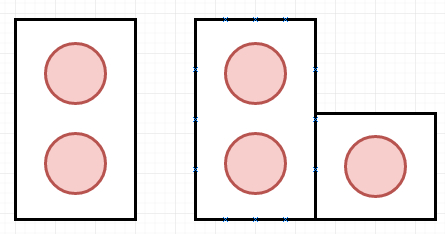# Program to count number of configurations are there to fill area with dominos and trominos in C++

Suppose we have two shapes, Domino and Tromino. Dominos are 2 x 1 shape and Trominos are ‘L’ like shape. They can be rotated like below −If we have a number n, we have to find number of configurations to fill a 2 x n board with these two types of pieces. As we know in tiling, every square must be covered by a tile.

So if the input is 3, then the output will be 5. So the arrangements can be [XYZ XXZ XYY XXY XYY] and [XYZ YYZ XZZ XYY XXY], here different letters are used for different tiles.

To solve this, we will follow these steps −

• Make an array called dp of size N + 5, set dp := 1, dp := 2 and dp := 5

• for i in range 4 to N

• dp[i] := 2*dp[i − 1] + dp[i − 3]

• return dp[N]

Let us see the following implementation to get better understanding −

## Example

Live Demo

#include <bits/stdc++.h>
using namespace std;
const int MOD = 1e9 + 7;
return ((a % MOD) + (b % MOD)) % MOD;
}
class Solution {
public:
int solve(int N) {
vector <int> dp(N + 5);
dp = 1;
dp = 2;
dp = 5;
for(int i = 4; i <= N; i++){
dp[i] = add(2 * dp[i − 1], dp[i − 3]);
}
return dp[N];
}
};
main(){
Solution ob;
cout << (ob.solve(3));
}

## Input

3

## Output

5

Updated on: 25-Dec-2020

44 Views